# Various POVRay Related Material

PovRay for scientific illustration: diagrams to photo-realism. POVRay density (DF3) files: Using POVRay as a volume renderer. Frustum clipping polygonal models for POVRay. CSG modelling, bump maps, media, quality settings, texture billboarding, fog, lens types, QuickTime VR navigable objects. Representing WaveFront OBJ files in POVRay.

# PovRay for scientific illustration: diagrams to photorealism

Written by Paul Bourke
May 2006

The following is a short illustration of how PovRay (a free and powerful rendering engine) can be used to create images suitable for all stages of scientific research: investigation, diagrams for papers, and high quality images for promotional purposes. The three versions below are all described identically with regard to their geometry, the differences are only in how that geometry is rendered. Note the PovRay files provided here are only intended to illustrate the rendering modes, there are external (#included) files and textures not included.

Figure 1 - the triptych [figure1.pov]

This is the diagram as it appeared in the journal, almost all features of PovRay are turned off, it is flat shaded, one light source at the camera position, no shadows, and the camera employs an orthographic projection. This is in the style typical of scientific or mathematical illustration.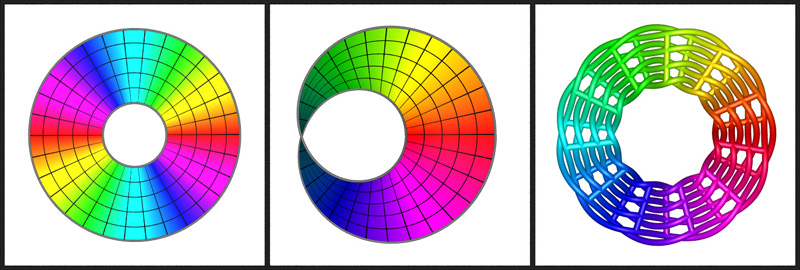Self-organization of synapses to form local maps. Left: polar plane representation of activity in an efferent field. Angles 0-2pi are represented by the colours of the spectrum, repeated twice. Middle: input map; saturation of synapses projected to the afferent field forms a Möbius projection from the efferent field. Distance relations are preserved, but angular relations are doubled to 0-4pi. Right: local map; synapses within the afferent field become saturated so as to form an intertwined mesh of bi-directional connections, closed over 0-4pi. [Figure legend from J.J. Wright et al. / Vision Research 46 (2006) 2703-2720]

Figure 2 [figure2.pov]

This is similar to the renderings of the geometry that were created during the scientific visualisation process. This is exactly the same geometry as in figure 1 above, the only difference is the rendering style, surface properties, lighting, and the placement of the components. Images of this form were used to solidify concepts in geometry and for discussion between researchers.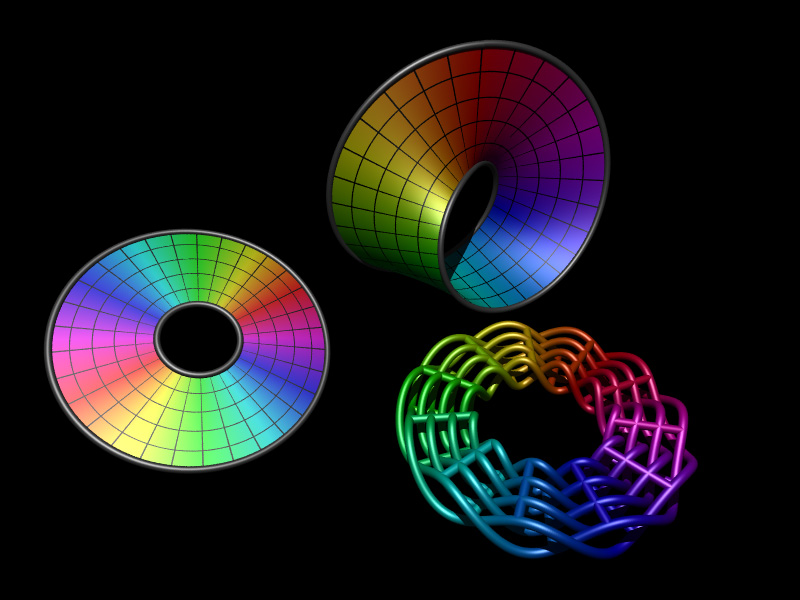Figure 3 [figure3.pov]

The following was created as a presentation form of the same geometry. It is rendered using radiosity (MegaPov), the lighting is defined by a HDR lighting environment of the staff tearoom (there are no other light sources). Additionally the result of the rendering was saved as a HDR image file (Radiance format) allowing exposure changes and filtering to be applied in post production without the usual artefacts that one encounters when using a limited 8 bits per r,g,b colour space. The page in the scene is how the diagram in figure 1 appeared in the draft version of the published paper. The final image was rendered at 6400 x 4800 pixels for a high resolution photographic print.Rapid prototype model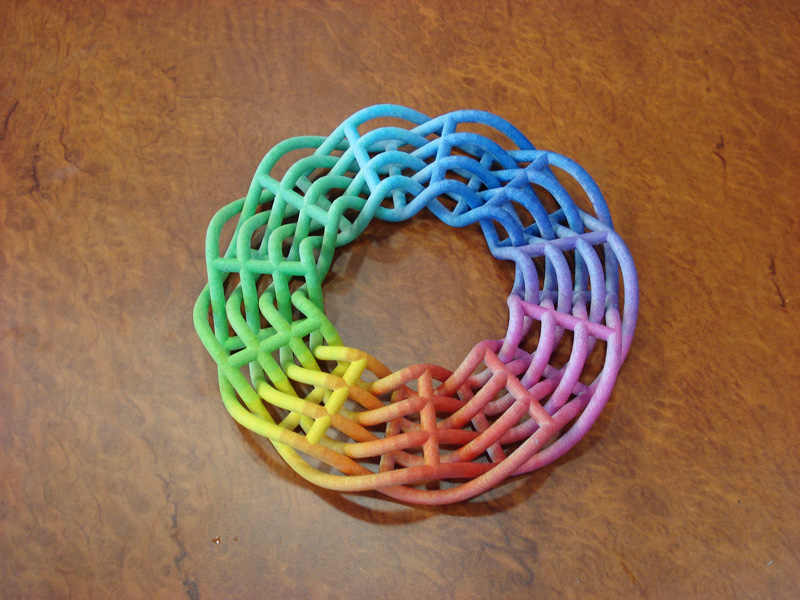# POVRay density (DF3) files

or

### Using POVRay as a volume renderer

Written by Paul Bourke
Original: June 2000, Updated: April 2003

A complete example using the MRI data illustrated on the right
mri.tar.gz
Render with: povray +Imri.pov mri.ini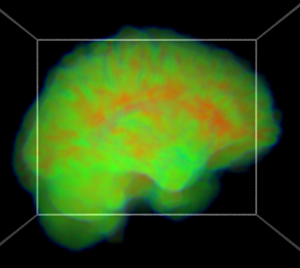POVRay, from version 3.1, supports a volumetric density media. This can be used to represent arbitrary media distributions, the volumetric values can be mapped to variations in density (using a density_map) or colour (using a colour_map). While this was primarily introduced as a powerful way to specify user defined media effects, it can also be used for certain volume rendering applications.

For a simple example, the following contains a number of Gaussian smeared points of different standard deviations. The POVRay file is here: example.pov, note that the density in this example just controls the emission in the media. While the density_map in this case is quite boring, different mappings are possible depending on the application. For more information on the media settings see the POVRay documentation.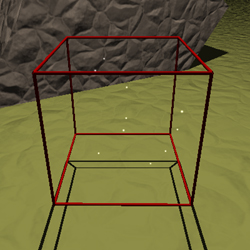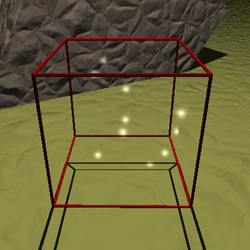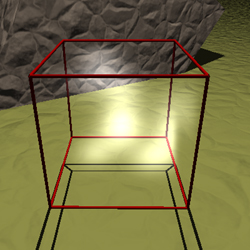A couple of simple examples adding a colour map are given below.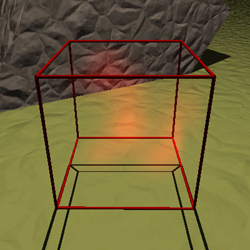```color_map { [0 rgb 0] [1 rgb <1,0,0>] }```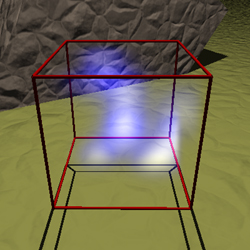```color_map { [0.0 rgb 0] [0.3 rgb <0,0,1>] [1.0 rgb 2] }```

By adding an absorption "absorption <1,1,1>" and turning on media interaction for the light sources, the media will cast shadows.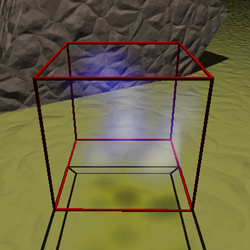```light_source { <0,5,0> color rgb <1,1,1> media_interaction on media_attenuation off } color_map { [0 rgb 0] [1 rgb <0,0,1>] }```

The format of a DF3 file is straightforward, it consists of a 6 byte header containing the 3 dimensions of the volume, this is followed by the voxel cells. Each voxel can be represented as a single byte (unsigned char), a 2 byte integer (unsigned short), or 4 byte integer (unsigned int). The 2 and 4 byte versions are written as big-endian (Intel chips are little endian, Mac PowerPC or G4/G5 are big-endian). POVRay works out which data type is used by the size of the file. The voxels are ordered as x varying the fastest, then y, and finally z varying the slowest.

The following gives the outline of how one might create a 3D volume and save it as a DF3 file where each voxel is a single unsigned byte. Using floats for the volume is simply to make the maths easier if one is creating arbitrary functions. For many applications it is OK to use short ints or even char directly. Note the endian of the short ints in the header and the order the volume voxels are written.

```   int i,j,k;
int nx=256,ny=256,nz=256;
float v,themin=1e32,themax=-1e32
float ***df3; /* So we can do easier maths for interesting functions */
FILE *fptr;

/* Malloc the df3 volume - should really check the results of malloc() */
df3 = malloc(nx*sizeof(float **));
for (i=0;i<nx;i++)
df3[i] = malloc(ny*sizeof(float *));
for (i=0;i<nx;i++)
for (j=0;j<ny;j++)
df3[i][j] = malloc(nz*sizeof(float));

/* Zero the grid */
for (i=0;i<nx;i++)
for (j=0;j<ny;j++)
for (k=0;k<nz;k++)
df3[i][j][k] = 0;

-- Create data volume data here --

/* Calculate the bounds */
for (i=0;i<nx;i++) {
for (j=0;j<ny;j++) {
for (k=0;k<nz;k++) {
themax = MAX(themax,df3[i][j][k]);
themin = MIN(themin,df3[i][j][k]);
}
}
}
if (themin >= themax) { /* There is no variation */
themax = themin + 1;
themin -= 1;
}

/* Write it to a file */
if ((fptr = fopen("example.df3","w")) == NULL)
return(FALSE);
fputc(nx >> 8,fptr);
fputc(nx & 0xff,fptr);
fputc(ny >> 8,fptr);
fputc(ny & 0xff,fptr);
fputc(nz >> 8,fptr);
fputc(nz & 0xff,fptr);
for (k=0;k<nz;k++) {
for (j=0;j<ny;j++) {
for (i=0;i<nx;i++) {
v = 255 * (df3[i][j][k]-themin)/(themax-themin);
fputc((int)v,fptr);
}
}
}
fclose(fptr);
```

If 2 or 4 byte voxels are being saved from a little-endian machine (Intel) then the following macros may be helpful.

```#define SWAP_2(x) ( (((x) & 0xff) << 8) | ((unsigned short)(x) >> 8) )
#define SWAP_4(x) ( ((x) << 24) | \
(((x) << 8) & 0x00ff0000) | \
(((x) >> 8) & 0x0000ff00) | \
((x) >> 24) )
#define FIX_SHORT(x) (*(unsigned short *)&(x) = SWAP_2(*(unsigned short *)&(x)))
#define FIX_INT(x)   (*(unsigned int *)&(x)   = SWAP_4(*(unsigned int *)&(x)))
```

So for example to write 2 bytes (unsigned short) then one have the writing loop as follows.

```   for (k=0;k<nvol;k++) {
for (j=0;j<nvol;j++) {
for (i=0;i<nvol;i++) {
v = 65535 * (df3[i][j][k]-themin)/(themax-themin);
FIX_SHORT(v);
fwrite(&v,2,1,fptr);
}
}
}
```
Further examples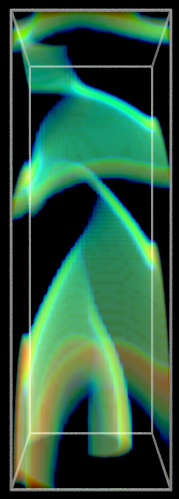```#declare NX = 32; #declare NY = 32; #declare NZ = 96; #declare theinterior = interior { media { intervals 100 ratio 0.5 samples 4,4 method 2 emission <1,1,1> / 10 absorption <1,1,1> / 30 scattering { 1, <0,0,0> } confidence 0.999 variance 1/1000 density { density_file df3 "x1d.df3" interpolate 1 color_map { [0.00 rgb <0,0,0>] [0.92 rgb <0,0,1>] [0.96 rgb <0,1,0>] [1.00 rgb <1,0,0>] } } } } box { <0,0,0>, <1,1,1> pigment { rgbf 1 } interior { theinterior } hollow translate <-0.5,-0.5,-0.5> scale } ```

MRI "brain on fire" rendering.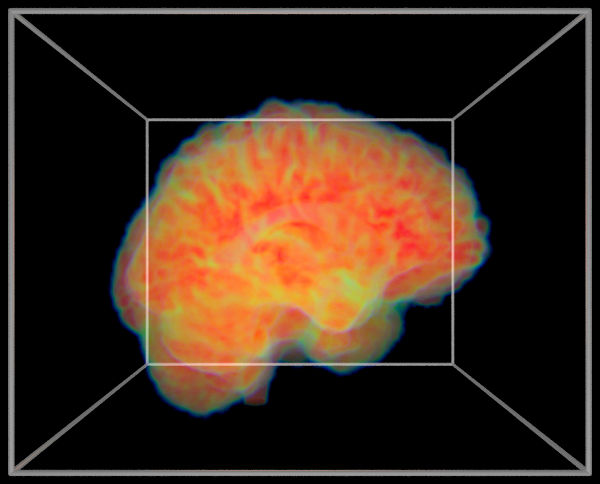Cosmological simulations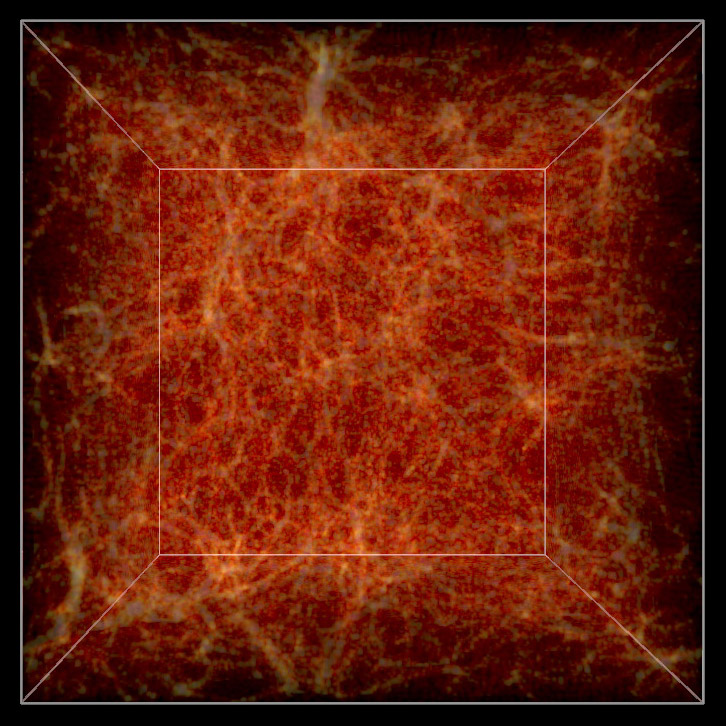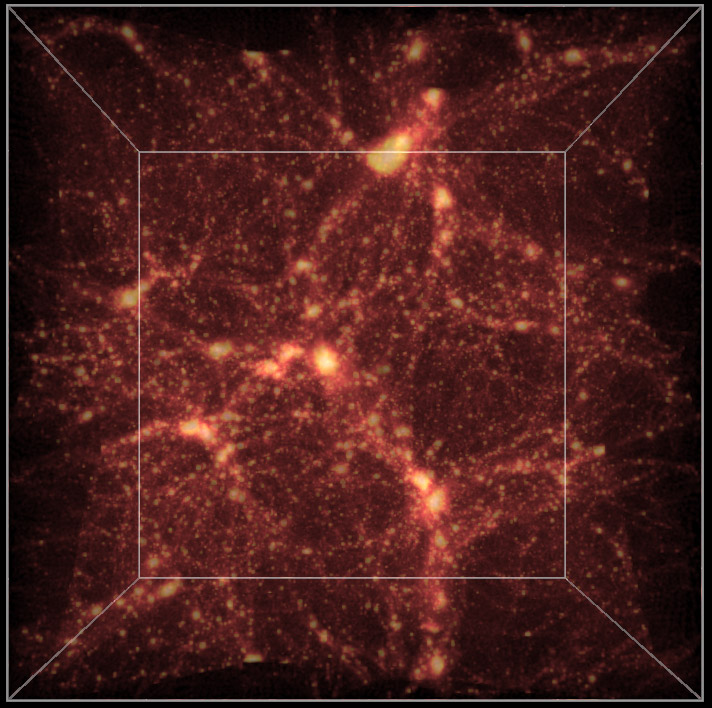# Frustum Clipping for PovRay

Written by Paul Bourke
May 2001

In normal rendering applications the potential exists for any piece of the geometry making up a scene to be visible or to have some effect on the visible part of the scene. For example, objects that can't be directly seen may be visible in reflective surfaces or they may cast shadows into the visible area.

It is however quite common in scientific visualisation projects that there is simply too much data for PovRay to render directly. One needs to get up to "tricks", for example, creating geometry at variable resolutions depending on the distance from the camera. Another trick that will discussed here is to prune away any geometry not directly visible. The particular rendering here involved a topology model of Mars containing over 130 million polygons. The polygonal approximation was adjusted depending on the distance of the camera to the surface to give about one polygon per pixel. In order to further reduce the polygons given to PovRay the polygons outside the view frustum were removed.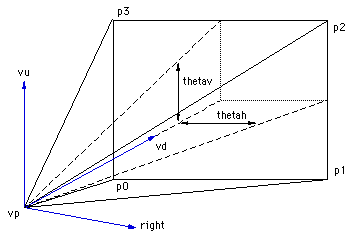In order to compute which polygons are outside the frustum one needs to be able to define the 4 planes making up the view frustum against which each vertex of the polygons will be tested. If one defines the horizontal aperture as thetah then the vertical aperture thetav is given by the following.

thetav = 2 * atan(HEIGHT * tan(thetah/2) / WIDTH)

Where WIDTH and HEIGHT are the image dimensions.

The points p0, p1, p2, p3 making up the corners of frustum can be computed using the C source below.

```   p0 = vp;
p0.x += vd.x - right.x*tan(thetah/2) - vu.x*tan(thetav/2);
p0.y += vd.y - right.y*tan(thetah/2) - vu.y*tan(thetav/2);
p0.z += vd.z - right.z*tan(thetah/2) - vu.z*tan(thetav/2);
p1 = vp;
p1.x += vd.x + right.x*tan(thetah/2) - vu.x*tan(thetav/2);
p1.y += vd.y + right.y*tan(thetah/2) - vu.y*tan(thetav/2);
p1.z += vd.z + right.z*tan(thetah/2) - vu.z*tan(thetav/2);
p2 = vp;
p2.x += vd.x + right.x*tan(thetah/2) + vu.x*tan(thetav/2);
p2.y += vd.y + right.y*tan(thetah/2) + vu.y*tan(thetav/2);
p2.z += vd.z + right.z*tan(thetah/2) + vu.z*tan(thetav/2);
p3 = vp;
p3.x += vd.x - right.x*tan(thetah/2) + vu.x*tan(thetav/2);
p3.y += vd.y - right.y*tan(thetah/2) + vu.y*tan(thetav/2);
p3.z += vd.z - right.z*tan(thetah/2) + vu.z*tan(thetav/2);
```

Where vp is the view position vector, vd is the unit view direction vector, vu is the unit up vector, and right is the unit vector to the right (the cross product between vd and vu). The 4 frustum planes are (vp,p0,p1), (vp,p1,p2), (vp,p2,p3), (vp,p3,p0) from which the normal of each plane can be computed.

A simple C function that determines which side of a plane a vertex lies might be as follows.

```/*
Determine which side of a plane the point p lies.
The plane is defined by a normal n and point on the plane vp.
Return 1 if the point is on the same side as the normal,
otherwise -1.
*/
int WhichSide(XYZ p,XYZ n,XYZ vp)
{
double D,s;

D = -(n.x*vp.x + n.y*vp.y + n.z*vp.z);
s = n.x*p.x + n.y*p.y + n.z*p.z + D;

return(s<=0 ? -1 : 1);
}
```

The example below shows a portion of the landscape rendered on the left. Moving the camera back a bit shows that the geometry (of the whole planet) has been clipped to remove any polygons not within the view frustum. Note that as well as frustum clipping, back facing polygons have also been removed.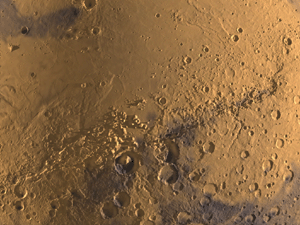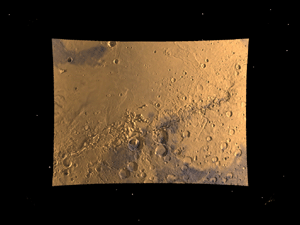# POVRAY CSG modelling

Written by Paul Bourke
February 1998

The following image and accompanying geometry file csg.pov illustrate the basics of the CSG (Constructive Solid Geometry) operations supported by POVRAY. The operations are performed on an intersecting circle and cylinder. Note that while the union and merge appear to give the same result, in the later the interior structure does not exist. This can be demonstrated by doing the rendering using a transparent material.# Bump Maps in PovRay

Written by Paul Bourke
April 2001

Bump maps are a way of creating the appearance of surface detail without needing to create additional geometric detail. This is achieved by perturbing the normals at each point on the surface. Since the normal is used to determine how light interacts with the surface the appearance of the surface is affected. The effect is very powerful, in some of the examples below it is hard to imagine that the surface hasn't been geometrically modified.
The following illustrate some of the procedural bump maps provided by PovRay. Not all the bump maps are shown but the more interesting ones are. This gives a quick visual index when looking for a particular effect. Note that some bump maps create artefacts on the curved surface used below, this is because they are designed for planar surfaces.

 agate ```#declare thenormal = normal { agate agate_turb 0.5 scale 1 } ```bozo previously known as spotted ```#declare thenormal = normal { bozo scale 0.1 } ```brick ```#declare thenormal = normal { brick brick_size 1 mortar 0.02 scale 1 } ```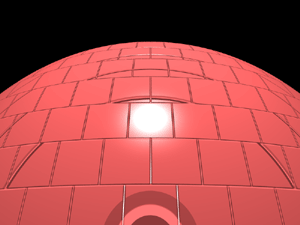bumps ```#declare thenormal = normal { bumps scale 0.2 } ```checker ```#declare thenormal = normal { checker 0.75 scale 0.6 } ```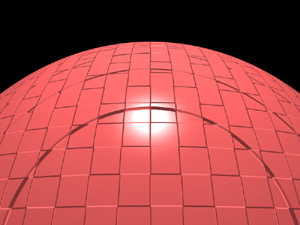crackle ```#declare thenormal = normal { crackle 1 scale 0.5 } ```dents ```#declare thenormal = normal { dents 1 scale 0.4 } ```gradient ```#declare thenormal = normal { gradient z,1 scale 0.5 rotate <0,45,0> } ```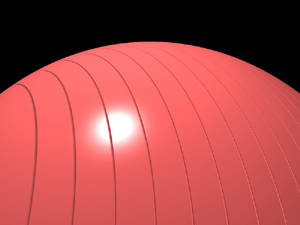granite ```#declare thenormal = normal { granite scale 1 } ```hexagon ```#declare thenormal = normal { hexagon 1 scale 0.1 } ```leopard ```#declare thenormal = normal { leopard 1 scale 0.1 } ```mandel ```#declare thenormal = normal { mandel 200,0.5 scale 2 rotate <0,90,0> rotate <0,0,15> } ```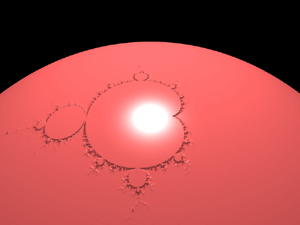marble ```#declare thenormal = normal { marble 1 scale 0.2 rotate <0,45,0> } ```quilted ```#declare thenormal = normal { quilted 1 control0 0.5 control1 0.5 scale 0.75 } ```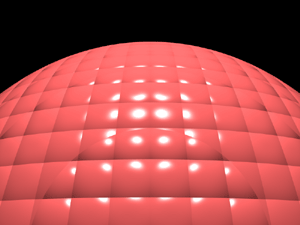radial ```#declare thenormal = normal { radial 1 frequency 200 rotate <70,0,0> rotate <0,45,0> } ```ripples ```global_settings { number_of_waves 10 } #declare thenormal = normal { ripples 1 frequency 10 phase 0 translate <-0.5,-0.5,-0.5> scale 1 rotate <0,0,45> } ```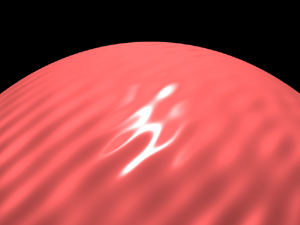spiral1 ```#declare thenormal = normal { spiral1 2,1 scale 1 rotate <45,0,0> } ```spiral2 ```#declare thenormal = normal { spiral2 1,1 scale 1 rotate <45,0,0> } ```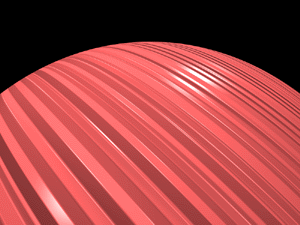waves ```global_settings { number_of_waves 10 } #declare thenormal = normal { waves 1 frequency 10 phase 0 translate <-0.5,-0.5,-0.5> scale 1 rotate <0,0,45> } ```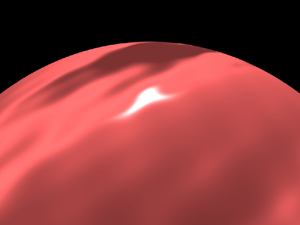wood ```#declare thenormal = normal { wood 1 scale 0.1 } ```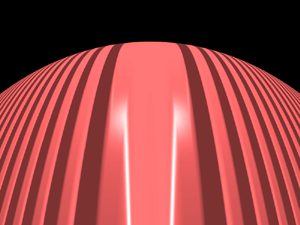wrinkles ```#declare thenormal = normal { wrinkles 1 scale 0.6 } ```Scene file: bump.pov ```#declare VP = <15,0,0>; #declare VD = <-1,0.5,0>; #declare VU = <0,1,0>; camera { location VP up y right -4*x/3 angle 60 sky VU look_at VP+VD } global_settings { ambient_light rgb <1,1,1> } background { color rgb <0,0,0> } light_source { VP + 10*VU color rgb <1,1,1> } #declare thefinish = finish { ambient 0.5 diffuse 0.7 specular 0.4 roughness 0.001 phong 1 } sphere { <0,0,0>, 10 pigment { color rgb <250/255.0,100/255.0,100/255.0> } finish { thefinish } normal { thenormal } } ``` ini file: bump.ini ```Input_File_Name=bump.pov Output_File_Name=bump.tga Output_File_Type=T Buffer_Output=off Width=800 Height=600 Antialias=on Antialias_Threshold=0.01 Statistic_Console=off Warning_Console=off Debug_Console=off Display=on ```

# Media (PovRay 3.5)

Written by Paul Bourke
August 2002
 Introduction The use of media within PovRay to achieve a particular effect can be challenging. The process is generally one of repeated trial and error where the result of a parameter change mostly hard to predict. The following attempts to illustrate a range of effects, hopefully as a starting point for the readers own exploration. Scene files test.ini -- test.pov The test scene consists of a unit sphere resting on a ground surface, this sphere will contain the media. There are two white lights, one directly above the sphere and the other closer to the centre of the sphere. In order to observe the effect of the media on objects, cylinders are placed behind the media and spheres are placed through the centre of the media sphere. Media sphere The media in these examples is bound within a sphere. The density is varied radially using the "spherical" pattern modifier which returns 1 in the centre and 0 at a radius greater than 1. The sphere below has all the media attributes (scattering, emission, and absorption) set to zero, effectively so the media has no effect. The resulting rendering is on the right. ```sphere { <0,0,0>, 1 hollow texture { pigment { rgbt <1,1,1,1> } finish { ambient 0 diffuse 0 } } interior { media { intervals 30 ratio 0.9 samples 2,4 confidence 0.9 variance 1.0/128.0 method 1 absorption <0.0,0.0,0.0> emission <0,0,0> scattering { 1, <0,0,0> extinction 0 } density { spherical turbulence 0 density_map { [0.0 color rgb 0 ] [1.0 color rgb 1 ] } } } } translate <0,0,1> } ``` Scattering, absorption, emission Three renderings on the right illustrate setting non-zero absorption, emission, and scattering. In each case there is no colour variation, so white light is scattered, white light is emitted, or white light is absorbed. Note that in the absorbing case the light passing through the media sphere from the light above ends up being reddish. A word of warning, scattering media can be very CPU expensive. Multiple density Multiple densities result in the densities being multiplied together (compared to multiple media where the densities are added together). In this example the goal was to have a slow transition from high to 0 density but to have turbulent variation within the media, this can be achieved with the following density description. ``` density { spherical density_map { [0.0 color 0 ] [1.0 color 1 ] } } density { bozo scale 0.2 density_map { [ 0.0 color 0.1 ] [ 1.0 color 1 ] } } ``` This can be animated by using a function of the clock variable with phase and turbulence. ``` density { bozo scale 0.2 turbulence 1 phase clock density_map { [ 0.0 color 0.1 ] [ 1.0 color 1 ] } } ``` Colour variation Colour variation can be added throughout the media by adding a colour map. Because there are two density sections the effects are multiplied together, the result modulated by the amount of scattering, emission, and absorption. The following colour map creates a green central region (spherical = 1) and red rim (spherical approaching 0). ``` density { spherical density_map { [ 0.0 color 0 ] [ 1.0 color 1 ] } } density { spherical color_map { [ 0.0 color <1,0,0> ] [ 1.0 color <0,1,0> ] } } ```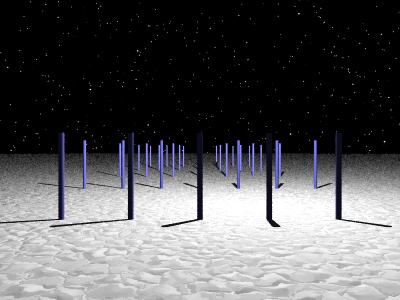0 absorption, 0 scattering, 0 emission1 absorption, 0 scattering, 0 emission0 absorption, 0 scattering, 0.5 emission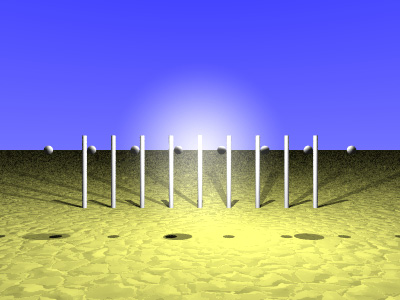0 absorption, 0.25 scattering, 0 emissionblue absorption, 0 scattering, 0 emission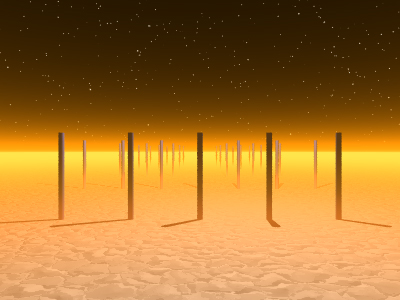Multiple media, emission only and bozo patternColour map, green to red spherical ramp, emission only

# POVRAY quality settings

Written by Paul Bourke
June 1999, updated for version 3.6 Oct 2006

The following illustrates renderings from POVRAY using the different quality settings. The scene being rendered for this example is quality.pov. The quality setting in POVRAY is set by using +Qn on the command line or Quality=n in a ".ini" file. All the rendering below were done with antialiasing on (otherwise default antialiasing settings) and rendered at 400x400, the relative times are multiples of the quality=1 raytracing time.

 Quality=1 Quick colours and ambient light Time unit = 1Quality=3 Calculate specific diffuse and ambient light Time unit = 1.6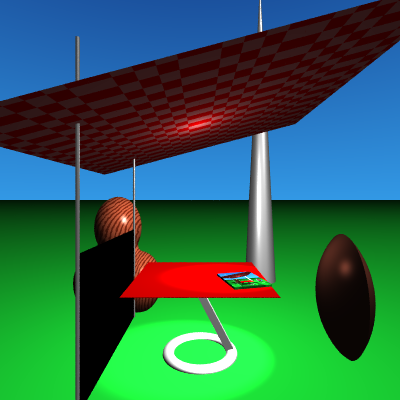Quality=5 Include shadows and extended lights Time unit = 2.4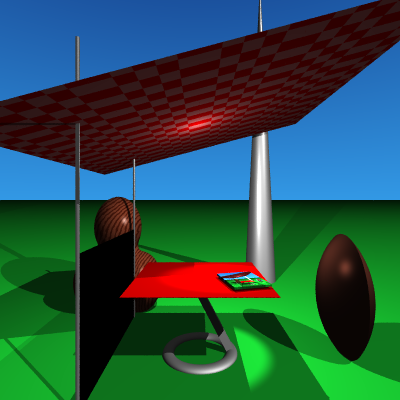Quality=7 Include texture patterns Time unit = 3.0Quality=9 Calculate reflected, refracted, and transmitted light Time unit = 7.2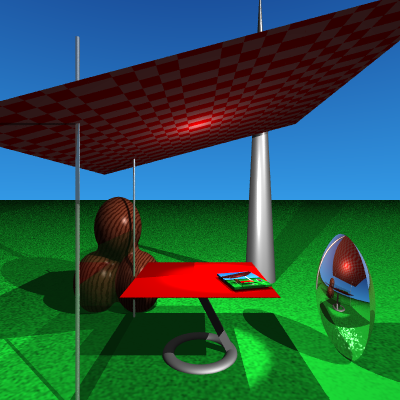# Texture billboarding in PovRay

Written by Paul Bourke
May 2002

Billboarding is a well established technique that maps a texture onto a planar surface that stays perpendicular to the camera. It is commonly used in interactive OpenGL style applications where textures are a much more efficient means of representing detail than creating actual geometry. The classic example is to represent pine trees (relatively radially symmetric), the texture image of the tree always faces the camera giving the impression of a 3D form.

The example that will be used here is the creation of a galaxy that rotates slowly and stays facing a camera that moves along a flight path. For each section and image below a PovRay file is provided which illustrates the step and can be used to create the image. path.

PovRay default texture coordinates (default.pov)

The texture will be mapped onto a disc, used because the galaxy images were mostly circular, a polygon could just as easily have been used. The default PovRay texture lies on the x-y plane at z=0 as shown below.Changing the orientation (orientate.pov)

The first step is to consider how to transform the texture so that it faces the camera. The camera model used here is as follows, where normally the camera position (VP), camera view direction (VD), and up vector (VU) are set by the flight path description.

```camera {
location VP
up y
right -4*x/3
angle 60
sky VU
look_at VP + VD
}
```

The view direction, up vector, and right vector need to be kept mutually perpendicular, to be more precise, orthonormal. The PovRay transform statement is used to orientate the texture coordinate system so it is perpendicular to the camera view direction.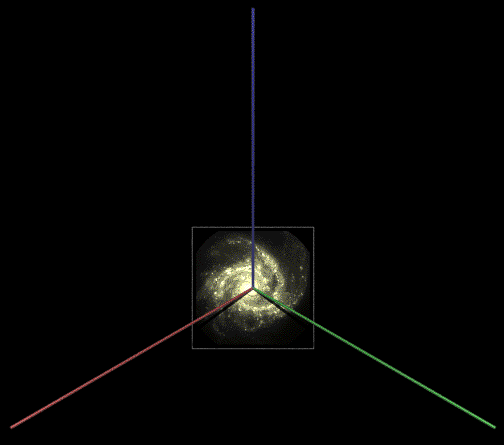Changing the position (position.pov)

In the above the disc and texture are still centered on the origin so they now need to be translated to the correct position. This could be done in the transform above (see last row of zeros) but a separate translate has been used here.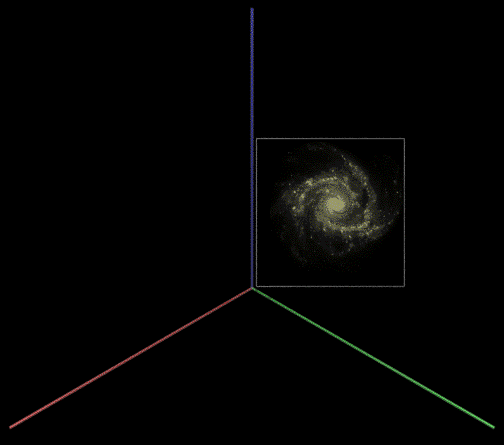Changing the orientation (final.pov)

Finally, the rotation is done, one only has the ensure that it's done at the right stage, namely while the galaxy was still centered at the origin.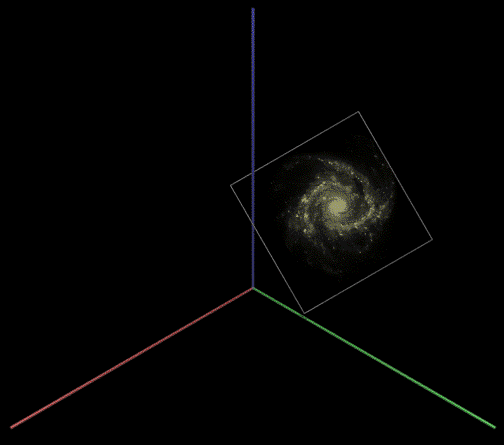# Fog (PovRay 3.5)

Written by Paul Bourke
August 2002
 Scene files fog.ini -- fog.pov The scene on the right was created in order to test the effect of various fog types and variable. Constant fog The simplest type of fog (type 1) is uniform in all directions, the rate at which the fog colour alters the colour of objects is proportional to the exp(-d/d0) function where d0 is the argument in the "distance" variable. So, if the object is d0 away the colour contribution for that pixel will be 0.63 of the fog colour plus 0.37 of the object colour. An object twice d0 away the colour will be 0.86 of the fog colour and 0.14 of the object colour. ```fog { /* Range for exp(-1) colour contribution */ distance 6 color rgb <1,0.6,0> fog_type 1 } ``` Transmittance and Filter The transmittance and filter of the fog colour is used to set the minimum translucency and the degree of filtering of light passing through the fog. So a transmittance of 0 and filter of 0 (eg: colour rgbft <1,0.6,0,0,0>) would give the same result as "colour rgb <1,0.6,0>". Increasing the filtering means the light sources become increasingly coloured by the fog, this in turn will affect the colour of objects illuminated by the light. Increasing the transmittance sets an upper limit on the degree to which the fog blocks distant objects. An example of the right show the result with a 50% filtering. ```fog { distance 6 color rgbft <1,0.6,0,0.5,0> fog_type 1 } ``` and a 50% transmittance. ```fog { distance 6 color rgbft <1,0.6,0,0,0.5> fog_type 1 } ``` Ground Fog PovRay has a second type of fog (type 2) called "ground fog", this has a vertical density dependence. Control of the vertical dependence is made with two variables, the first (fog_offset) sets a height below which the fog has a constant density, the second variable (fog_alt) controls the rate of density falloff above that height. A small value of "fog_alt" compared to "fog_offset" results in a sharp transition. The exact equation for heights above the fog_offset is (1 + (height - fog_offset) / fog_alt)-2 So at a height of fog_alt above the fog_offset the fog density is 1/4 of what it is at (or below) fog_offset. At twice fog_alt above fog_offset the density is 1/9th. ```fog { distance 6 color rgbft <1,0.6,0,0,0> fog_type 2 up <0,0,1> fog_offset 0.5 /* Constant below this */ fog_alt 0.5 /* Decay rate */ } ``` Turbulence The turbulence variable is used the same as when applied to patterns, the argument dictates the degree of turbulence. An additional variable "turb_depth" determines where along the ray the turbulence is calculated. 0 indicates at the camera, 1 is at the first object the traced ray strikes. The other parameters for turbulence such as octaves, lambda, and omega can be specified to control the turbulence function. ```fog { distance 6 color rgbft <1,0.6,0,0,0> fog_type 2 up <0,0,1> fog_offset 0.5 fog_alt 0.5 turbulence 2 turb_depth 0.9 } ``` Multiple fog Multiple fogs may be specified, the effect is additive. Here is a sharp yellow layer under a brownish top layer. ```fog { distance 6 color rgbft <1,0.6,0,0,0> fog_type 2 up <0,0,1> fog_offset 0.5 fog_alt 0.5 } fog { distance 6 color rgbft <0.8,0.3,0.3,0,0> fog_type 2 up <0,0,1> fog_offset 0.3 fog_alt 0.1 } ``` Night vision Limited night vision can be simulated with black fog. ```fog { distance 6 color rgbft <0,0,0,0,0.3> fog_type 1 } ```No fog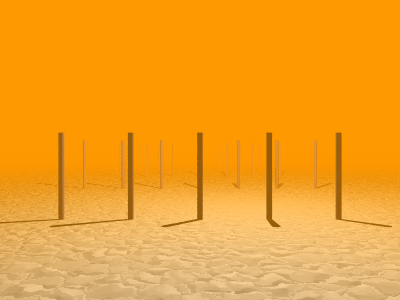Constant fog with no transmittance or filtering.Constant fog with 50% filtering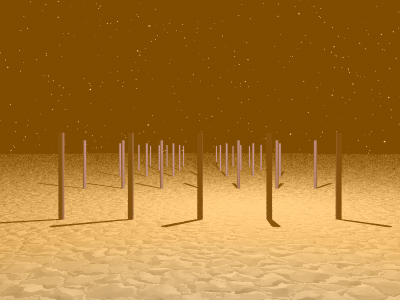Constant fog with 50% transmittance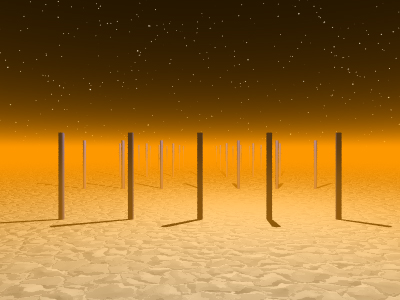Ground fogTurbulenceMultiple layers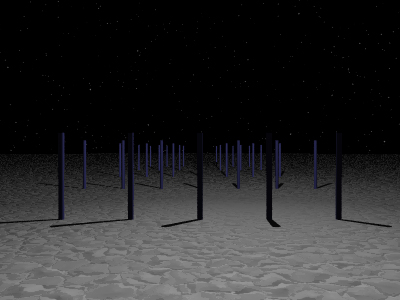Limited night vision

# PovRay lens types

Written by Paul Bourke

AutoCAD Model courtesy of Stephanie Phan, Andrew Hutson, Frank Sears from the Melbourne University School of Architecture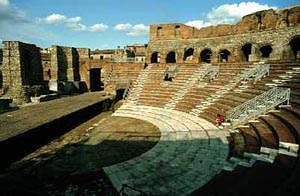The lens types available in version 3 of PovRay are illustrated here along with the camera specifications for each one. The way (and order) in which PovRay treats the various camera attributes isn't always obvious, the section of the manual dealing with the camera settings should be read carefully.

A model of the Roman Beneventum stadium has been used to illustrate the various lens types. The geometry for the model was imported into MicroStation and converted into a PovRay scene through the WRL export, this creates the cleanest and most convenient geometry output of all the formats provided by AutoCAD and MicroStation.

The plans and elevations of this model are given on the right. A rendering using a standard perspective projection (aperture=60) is shown below.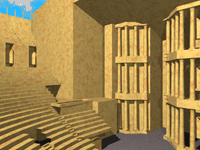Front viewTop view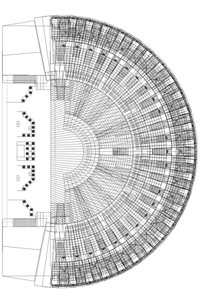Left view

 Perspective The camera aperture for the following is 90, 120, and 150. The model used by PovRay is that of a straightforward pinhole camera, that is, there are no lens effects. The "angle" argument is the preferred method of specifying the camera aperture, in earlier version of PovRay the relative length of the "direction" and "right" vector determined the camera aperture. ```#declare VP = <0,12000,30000>; #declare VD = <1,0,1>; #declare APERTURE = 120; camera { perspective location VP up y right -4*x/3 angle APERTURE sky <0,1,0> look_at VP+VD } ``` The same camera position and view direction (VP and VD) will be used for the remainder of the renderings unless otherwise specified. Note the negative value for the right vector, this changes the coordinate system from a left to a right hand one (this is what the modelling software used). The 4/3 scale factor for the right vector creates the correct aspect ratio for an image size of 800x600 as specified in the ini file. ```Width=800 Height=600 ```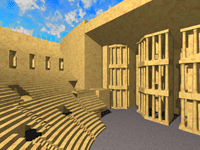Fisheye A fisheye lens implements a standard spherical projection, the "angle" may range from 0 to 360 degrees. At 180 degrees half the visible space is visible, at 360 degrees the whole visual space is visible (note that in this case a point behind the viewer is stretched around the perimeter of the projection circle). The fisheye lens examples here correspond to camera apertures of 180, 270, and 360. ```camera { fisheye location VP up y right -x angle APERTURE sky <0,1,0> look_at VP+VD } ``` This projection is normally created with a 1:1 aspect ratio so that the projection results in a circular image.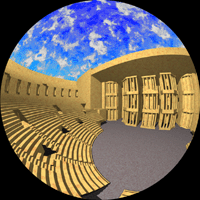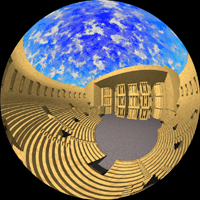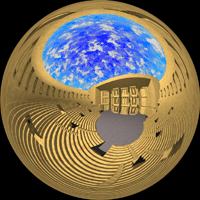Ultra wide angle This projection type is related to the fisheye projection except the resulting image is mapped onto a rectangular area instead of a circular one. The examples here are for 180 and 270 degrees. ```camera { ultra_wide_angle location VP up y right -x angle APERTURE sky <0,1,0> look_at VP+VD } ```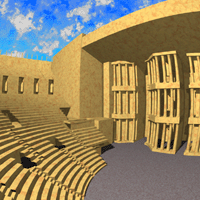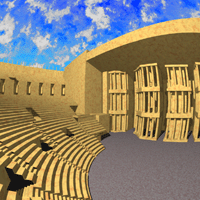Omnimax This is a special purpose projection for Omnimax theatres. The angle is fixed at 180 degrees. Normally Omnimax images/movies are filmed with a matching lens system to the intended projection system, the projection system "undoes" the fisheye like distortion introduced with the matching camera. ```camera { omnimax location VP up y right -x angle 180 /* Not used */ sky <0,1,0> look_at VP+VD } ``` Comparing this projection with the 180 degree fisheye, the top half appears the same but the bottom half has been elliptically clipped.Panoramic This is also known as a cylindrical equirectangular projection. ```camera { panoramic location VP up y right -2*x angle APERTURE sky <0,1,0> look_at VP+VD } ```Cylindrical For this projection the scene is mapped onto a cylindrical band, PovRay allows the band to run vertically as well as horizontally. PovRay also supports two modes, one where the view point remains in the same place and another where the view point moves around the cylinder. ```camera { cylinder 2 location VP up y right -2*x angle 180 sky <0,1,0> look_at VP+VD } ```Cuberender This is a mapping external to PovRay, further details can be found here. ```camera { perspective location VP up y right -x angle 90 #switch (clock) #case (1) sky <0,1,0> look_at VP +x #break #case (2) sky <0,1,0> look_at VP -z #break #case (3) sky <0,1,0> look_at VP -x #break #case (4) sky <0,1,0> look_at VP +z #break #case (5) sky <1,0,0> look_at VP +y #break #case (6) sky <1,0,0> look_at VP -y #break #end } ```# Making QuickTime Navigable objects using PovRay

Written by Paul Bourke
April 2000

QuickTime VR navigable objects are one of the original features (along with panoramic) Apple built into QuickTime after simple movie playing. They allow exploration of an object by moving a virtual camera around the object, generally on the surface of a sphere. Internally they are just a linear QuickTime movie but with some extra information to indicate which frame sets form the lines of longitude and latitude.

The three steps in creating a QT navigable object are as follows:

• 1.  Create the frames in the correct order.

• 2.  Build a QuickTime movie from these frames. This is normally done using QuickTime Pro but there are other software solutions a well. Any QuickTime codec can be used to compress this movie.

• 3.  Add the extra information that instructs a QuickTime player that this is a navigable object and how the lines of latitude and longitude are sampled. There are some tools from Apple (and others) that will do this, I used something called "Make QTVR Object" from the "QuickTime VR Dev Kit".

The rest of this document will describe how to perform the first step above using PovRays animation support based upon the clock variable, namely, creating all the images required and in the correct order. In polar coordinates (R,Theta,Phi), sometimes called spherical coordinates, given a fixed radius (R) all the points lie on a sphere. The two angles (Theta,Phi) determine the lines of longitude (0 to 360) and latitude (90 to -90) respectively.These lines of latitude and longitude can be "unwrapped" from the sphere and represented as a grid.QuickTime VR object movies allow any rectangular part of this grid to be used. In most cases the entire grid is used in which case panning right past theta=360 will wrap to theta=0 for any line of latitude. Note that the line along the top and bottom edge of the grid map to a single point at the north and south pole. Whichever part of the grid is used one will need to give the longitude and latitude bounds to the software that performs step 3 above.

In the following example using PovRay the object to be explored is assumed to be located at the origin, if this isn't the case it can readily be translated there or the PovRay code below modified to create the views about some other position. In the first section given below, the parameters that determine the grid resolution and range are specified. In the example here the resulting navigation will be in 5 degree steps left/right (longitude) and 10 degree steps up/down (latitude).

```/*
The ini file should have NLONGITUDE * (NLATITUDE + 1) frames
And a clock variable that goes across the same range
for example for NLONGITUDE = 72 and NLATITUDE = 18
Initial_Frame = 0
Final_Frame   = 1367
Initial_Clock = 0
Final_Clock   = 1367
Fill in the next 7 parameters
*/
#declare NLONGITUDE   = 72;
#declare NLATITUDE    = 18;
#declare LONGITUDEMIN = 0;
#declare LONGITUDEMAX = 360;
#declare LATITUDEMIN  = -90;
#declare LATITUDEMAX  = 90;
```

Next we compute the polar coordinates (theta,phi) with a constant camera range given by CAMERARADIUS above. From these the camera position (VP), view direction (VD), and up vector (VU) are derived.

```/* Calculate polar coordinates theta and phi */
#declare DLONGITUDE = LONGITUDEMAX - LONGITUDEMIN;
#declare DLATITUDE  = LATITUDEMAX - LATITUDEMIN;
#declare THETA = LONGITUDEMIN + DLONGITUDE * mod(int(clock),NLONGITUDE) / NLONGITUDE;
#declare PHI   = LATITUDEMAX - DLATITUDE * int(clock/NLONGITUDE) / NLATITUDE;
#if (PHI > 89.999)
#declare PHI = 89.999;
#end
#if (PHI < -89.99)
#declare PHI = -89.999;
#end
#debug concat("\n****** Clock: ",str(clock,5,1),"\n")
#debug concat("       Theta: ",str(degrees(THETA),5,1),"\n")
#debug concat("       Phi:   ",str(degrees(PHI),5,1),"\n")

/* Calculate the camera position */
#declare VP = CAMERARADIUS * <cos(PHI) * cos(THETA),cos(PHI) * sin(THETA),sin(PHI)>;
#declare VD = -VP;
#declare VU = <0,0,1>;
#declare RIGHT = vcross(VD,VU);
#declare VU = vnormalize(vcross(RIGHT,VD));
```

In the above the tweaking of PHI at 90 and -90 (the poles) is a nasty solution to the problem of creating the correct up vector (VU) at the poles, there are more elegant ways but this seems to work OK. This method works in combination with the cross product to calculate a right vector and then the correct up vector that is at right angles to the view direction.

These camera variables (position, view direction and up vector) are finally combined into a camera definition which may look something like the following.

```camera {
location VP
up y
right x
angle 60
sky VU
look_at <0,0,0>
}
```

An unfortunate reality of these navigable object movies is their size. For D1 degree steps in longitude and D2 latitude, the total number of frames for a full sphere is given by ((180+D2)/D2)*(360/D1). So for the example above D1 = 5 and D2 = 10 degrees so there are 1368 frames. For a smoother sequence where there 2 degree steps in both directions there would be 16380 frames! For this reason I haven't included an actual QuickTime VR object example movie.

Screen shot examples of various QT VR tools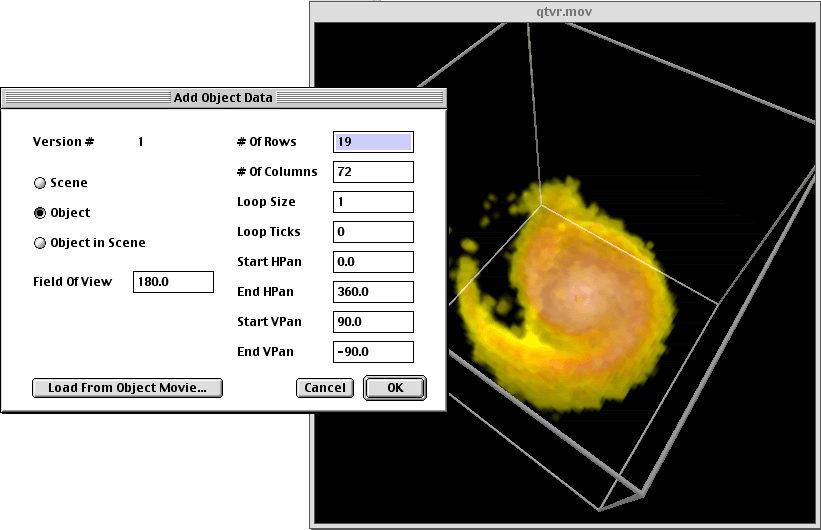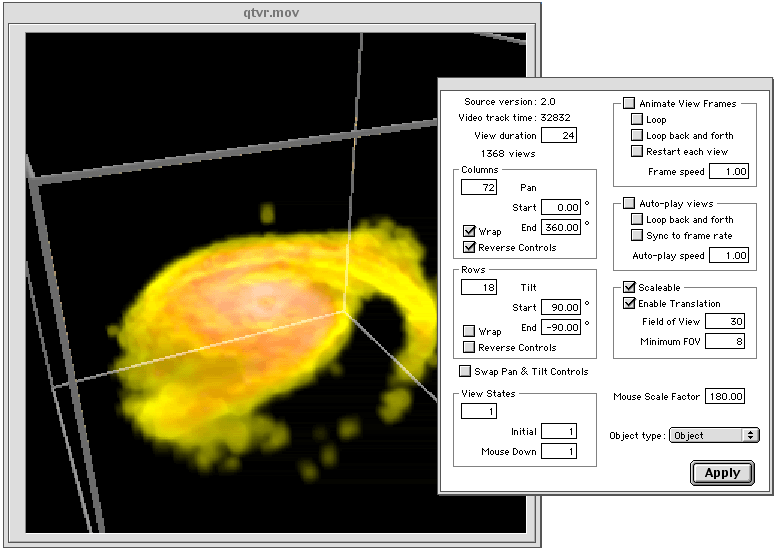# Representing WaveFront OBJ files in POVRay

Written by Paul Bourke
October 2012

With the introduction of the mesh2 primitive in POVRay there is now a nice one to one mapping between textured mesh files in WaveFront OBJ format and POVRay.

A textured mesh in OBJ format may be represented as follows, note that long lists of vertices, normals, etc have been left out in the interests of clarity. There are essentially 4 common sections, "v" are vertices, "vn" are normals, usually one per vertex, "vt" texture uv coordinates on the range 0 to 1, and finally faces defined by indices into the vertex, normal, and texture lists.

```# a comment
mtllib some.mtl
v -27.369801 -16.050600 18.092199
v -27.171200 -14.097800 17.699499
- cut -
vn -2.350275 -1.812223 -1.067749
vn -5.281989 0.596537 -2.515623
- cut -
vt 0.000000 0.000000
vt 0.780020 0.805009
- cut -
usemtl materialname
f 2766/2/2766 2767/3/2767 2768/4/2768
f 2778/14/2778 2779/15/2779 2777/13/2777
- cut -
```

The mapping to a POVRay mesh2 object is as follows

```// a comment
mesh2 {
vertex_vectors { 83954,
<-27.369801,-16.050600,18.092199>,
<-27.171200,-14.097800,17.699499>,
- cut -
}
normal_vectors { 83954,
<-2.350275,-1.812223,-1.067749>,
<-5.281989,0.596537,-2.515623>,
- cut -
}
uv_vectors { 86669,
<0.000000,0.000000>,
<0.780020,0.805009>,
- cut -
}
face_indices { 54515,
<2765,2766,2767>,
<2777,2778,2776>,
- cut -
}
normal_indices { 54515,
<2765,2766,2767>,
<2777,2778,2776>,
- cut -
}
uv_indices { 54515,
<1,2,3>,
<13,15,13>,
- cut -
}
uv_mapping
texture { texturename }
}
```
Note

• The indices for OBJ start from 1 while the indices for mesh2 start from 0.

• The texture for an obj file is described in a material file, for example

```newmtl materialname
Ka 0.200000 0.200000 0.200000
Kd 1.000000 1.000000 1.000000
Ks 1.000000 1.000000 1.000000
Tr 1.000000
illum 2
Ns 0.000000
map_Kd some.jpg
```
In POVRay it might be

```#declare texturename = texture {
pigment { image_map { jpeg "some.jpg" once } }
finish { ambient 0.5 diffuse 1 specular 0 }
}
```